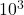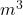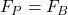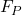## A small barge is being used to transport trucks across a river. If the barge is 10.00 m long by 8.00 m wide and sinks an additional 3.75 cm

Question

A small barge is being used to transport trucks across a river. If the barge is 10.00 m long by 8.00 m wide and sinks an additional 3.75 cm into the river when a loaded truck pulls onto it, determine the weight of the truck and load.

in progress 0
5 months 2021-08-13T01:43:41+00:00 1 Answers 28 views 0

1. Answer: Weight truck+load = 29.4×N

Explanation: When an object floats in a fluid, there is an upward force, caused by the liquid, acting on the object that opposes the weight of the immersed object. This force is called Buyoyant Force and is determined by:

B = d*V*g

where

d is density of the fluid;

V is volume of liquid displaced due to the immersed object;

g is acceleration due to gravity;

For the truck, the system is in equilibrium, which means buyoyant force is equal weight. Then:

Volume displaced is

V = 10*8*0.0375

V = 3Density of water: 1000kg/= 1000*3*9.8= 29.4×N

The weight of the truck and the load is 29.4×Newtons